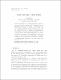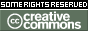Please use this identifier to cite or link to this item: `https://hdl.handle.net/10216/25719`
 Author(s): Christian Lomp Title: Duality for partial group actions Issue Date: 2008 Abstract: Given a finite group G acting as automorphisms on a ring A, the skew group ring A*G is an important tool for studying the structure of G-stable ideals of A. The ring A*G is G-graded, i.e. G coacts on A*G. The Cohen-Montgomery duality says that the smash product A*G#k[G]^* of A*Gwith the dual group ring k[G]^* is isomorphic to the full matrix ring M_n(A) over A, where n is the order of G. In this note we show how much of the Cohen-Montgomery duality carries over to partial group actions alpha in the sense of R.Exel. In particular we show that the smash product (A *_alpha G)#k[G]^* of the partial skew group ring A*_alpha G and k[G]^* is isomorphic to a direct product of the form K x eM_n(A)e where e is a certain idempotent of M_n(A) and K isa subalgebra of (A *_alpha G)#k[G]^*. Moreover A*_alpha G is shown to be isomorphic to a separable subalgebra of eM_n(A)e. We also look at duality for infinite partial group actions. Description: Given a finite group G acting as automorphisms on a ring A, the skew group ring A*G is an important tool for studying the structure of G-stable ideals of A. The ring A*G is G-graded, i.e. G coacts on A*G. The Cohen-Montgomery duality says that the smash product A*G#k[G]^* of A*Gwith the dual group ring k[G]^* is isomorphic to the full matrix ring M_n(A) over A, where n is the order of G. In this note we show how much of the Cohen-Montgomery duality carries over to partial group actions alpha in the sense of R.Exel. In particular we show that the smash product (A *_alpha G)#k[G]^* of the partial skew group ring A*_alpha G and k[G]^* is isomorphic to a direct product of the form K x eM_n(A)e where e is a certain idempotent of M_n(A) and K isa subalgebra of (A *_alpha G)#k[G]^*. Moreover A*_alpha G is shown to be isomorphic to a separable subalgebra of eM_n(A)e. We also look at duality for infinite partial group actions. Subject: Álgebra, MatemáticaAlgebra, Mathematics Scientific areas: Ciências exactas e naturais::MatemáticaNatural sciences::Mathematics URI: https://repositorio-aberto.up.pt/handle/10216/25719 Document Type: Artigo em Revista Científica Internacional Rights: openAccess License: https://creativecommons.org/licenses/by-nc/4.0/ Appears in Collections: FCUP - Artigo em Revista Científica Internacional

Files in This Item:
File Description SizeFormat
48110.pdfPublished paper200.1 kBAdobe PDFThis item is licensed under a Creative Commons License